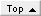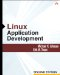# Section 19.1. Pseudo-Random Numbers

### 19.1. Pseudo-Random Numbers

Sometimes you want to provide the appearance of unpredictability, however. The C library contains functions for generating a well-respected series of pseudo-random numbers. These functions are easy to use and are the same on every Unix platform. Here is an example of how these functions are typically used:

` #include <stdlib.h> #include <time.h>...     srand(time(NULL) + getpid());     for (...; ...; ...) {         do_something(rand()); } `

It is common to seed the pseudo-random number generator with the current date, as returned by the time() function. time() returns the number of seconds since January 1, 1970, so the seed changes once per second and is therefore unique over a long time span (approximately 49,710 days on a 32-bit computer). If you want to keep a program from acting the same for two people who start it in the same second, add the current process ID to the time.

The numbers subsequently returned by the rand() function satisfy the mathematical property of random distribution but not of high entropy: For large enough samples, they are relatively well distributed within the space of possible 32-bit numbers, but it is possible to infer other numbers given one number. This means that these kinds of pseudo-random numbers are useful for almost every application that requests a random distribution of numbers. This includes games, Monte Carlo methods (here it is important to save the seed so that you or others can verify your results), and protocols that handle collision by inserting random delays.

Note that for debugging purposes, you may want to save the seed you used when you called srand() so that if a bug occurs that relies on data produced by the rand() function, you can use the same seed to produce the same stream of random numbers to reproduce the bug.Linux Application Development (paperback) (2nd Edition)
ISBN: 0321563220
EAN: 2147483647
Year: 2003
Pages: 168

Similar book on Amazon